# Laws of Illumination (Explanation And Formulas)

## The Inverse Square Law of Illuminance

This law states that the Illuminance (E) at any point on a plane perpendicular to the line joining the point and source is inversely proportional to the square of the distance between the source and plane.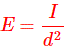Where, I is the luminous intensity in a given direction.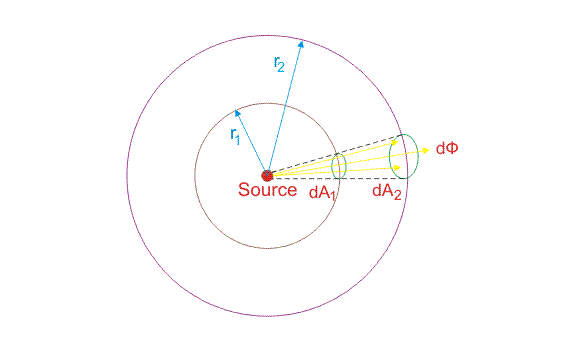Suppose a source is present with luminous intensity I in any direction. From this source two distances are taken as the radius making this source as centre.

As per the above figure, the two radiuses are r1 and r2. At distance r1 dA1 is the elementary surface area taken. In this direction of dA1, dA2 is considered at r2 distance.
dA1 and dA2 are within same solid angle Ω with same distributed luminous flux Φ.
Area dA1 at r1 receives the same amount of luminous flux as area dA2 at r2 as the solid are the same.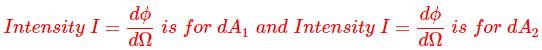Again solid angle for both elementary surfacesThe Illuminance at distanceThe Illuminance at distance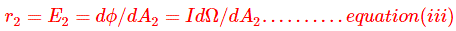Now, from equation (i) we get,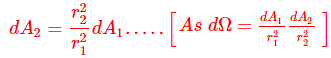Now in the equation (iii),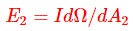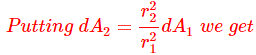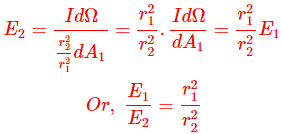This indicates the well known inverse square law relationship for point source.
It is seen that Illuminance varies inversely as the square of the illuminated point from the source.
If the light source is not a point source, then we can assume this large source as the summation of many point sources.
This relationship can be applied to all light sources.

### The Cosine Law of Illuminance

The law states that Illuminance at a point on a plane is proportional to the cosine of the angle of light incident (the angle between the direction of the incident light and the normal to the plane).It is the point source Illuminance equation.
Where, Iθ is the luminous intensity of the source in the direction of the illuminated point, Ɵ is the angle between the normal to the plane containing the illuminated point and the line joining the source to the illuminated point, and d is the distance to the illuminated point.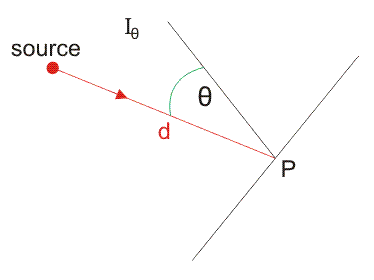But for non point source, the cosine law of Illuminance can be analyzed in term of luminous flux instead of luminous intensity.
The Illuminance or the surface density of the light flux received by an elementary area varies with the distance from the light source and the angle of the elementary area with respect to the direction of the light flux.
The maximum Illuminance occurs when the element of area receives the light flux normal to its surface.
When the element of area is tilted with respect to the direction of the light flux, the Illuminance or flux density on the elementary surface is reduced. This can be thought of in two ways.

1. The tilted elementary area (δA) cannot intercept all the light flux it previously received and so the Illuminance falls.
2. If the elementary area (δA) increases, the Illuminancefalls.For case (1) when the element δA is tilted by an angle Ɵ the amount of flux intercepted δA is given bySo the flux received by δA is reduced by a factor cosƟ.
Now the Illuminance at δA is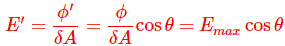For case (2) if all the flux intercepted by larger element δA’ :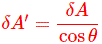So Illuminance becomes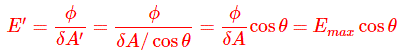Both cases of these approach result inWant To Learn Faster? 🎓
Get electrical articles delivered to your inbox every week.
No credit card required—it’s 100% free.